# Confidence Level Routine¶

Although the general, mass conservation, and semi-diffusive approaches can quickly provide confirmation of bistability for most examples, this may not always be the case. In fact, an important item of discussion is that these approaches cannot exclude bistability, even if a large amount of random decision vectors are explored. It is this uncertainty that we wish to address. This is done by assigning a probability that the minimum objective function value achieved is equal to the true global minimum. We achieve this probability by considering a slightly modified version of the unified Bayesian stopping rule in [BGS04] and Theorem 4.1 of [SF87], where the rule was first established.

Let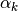and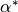denote the probability that the optimization routine has converged to the local minimum objective function value, say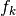, and global minimum objective function value, say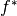. Assuming that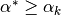for all local minimum valueswe may then state that the probability that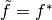is as follows: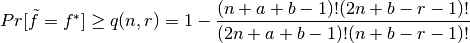,

where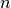is the number of initial decision vectors that are considered,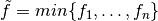,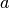and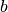are parameters of the Beta distribution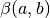, and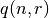is the confidence level. We then let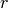be the number offor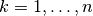that are in the neighborhood of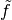.

Given our minimum objective function value is zero, for some networks it may be the case that theare nearly zero with respect to machine precision. For this reason, we say thatis in the neighborhood ofif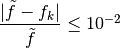.

This means thatis in the neighborhood ofif the relative error ofandis less than 1%. Ifis considered zero with respect to the system’s minimum positive normalized float, then we consider this value zero and provide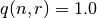, skipping the computation of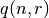. Thus, we can state that the probability that the obtainedis the global minimum (for the prescribed bounds of the decision vector) is greater than or equal to the confidence level. Using the standard practice in statistics, it should be noted that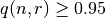is often considered an acceptable confidence level to make the conclusion thatis the global minimum of the objective function.

For information on how to enable the construction of a confidence level for each of the approaches, please refer to the following for each approach: